# SSAT Upper Level Math : Interpretation of Tables and Graphs

## Example Questions

1 3 Next →

### Example Question #1 : How To Read Tables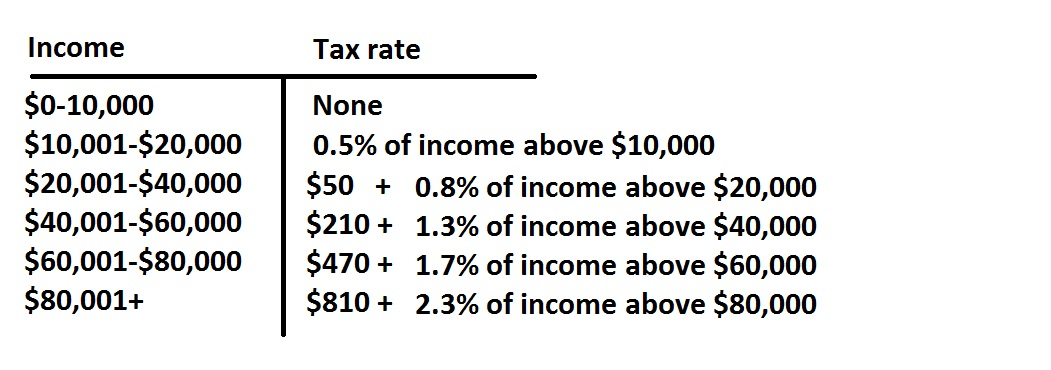The above is an annual income tax table for married couples for a given state.

Mr. Clarke earned a monthly salary of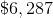throughout last year; Mrs. Clarke earned a monthly salary of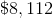, although she started on April 1. Also the couple claimed interest earnings of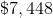and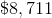in stock dividends. How much will the couple pay in income tax for that year (nearest dollar)?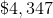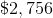The correct answer is not among the other choices.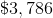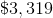Explanation:

Mr. and Mrs. Clarke earned a total of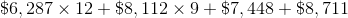(noting that Mrs. Clarke worked for nine months)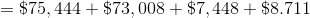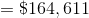This places them in the highest tax bracket, so they will pay $810 plus 2.3% of their income over$80,000: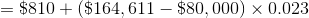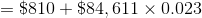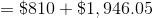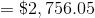### Example Question #1 : How To Read Tables

What is the probability of rolling a single die and it landing on 2 and 4?Explanation:

When you roll on die you can only get one possible value facing up.  The expression "the probability of getting a 2 and 4"  means that they both occur at the same time.  Since you can not get two results with one die, the probability must be.

### Example Question #1 : How To Read Tables

What is the probability of rolling a single die and it landing on 2 and 4?Explanation:

When you roll on die you can only get one possible value facing up.  The expression "the probability of getting a 2 and 4"  means that they both occur at the same time.  Since you can not get two results with one die, the probability must be.

### Example Question #1 : How To Read Tables

 Column1 Column2 1 0 2 0 3 2 4 3 5 4 6 5 7 8 8 8 9 8 10 8 11 5 12 0

Looking the the table given above, what is the range of the data set?Explanation:

The range of the data is the difference between the highest and lowest independent variable values.

In this set, the lowest isand the highest is.

The difference between these two is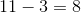.

### Example Question #1 : How To Read Tables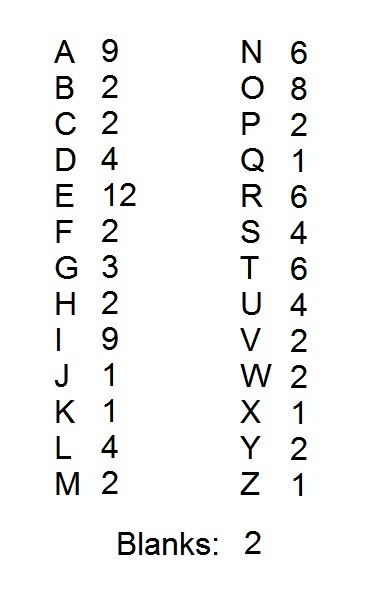A popular word game uses one hundred tiles, each of which is marked with a letter or a blank. The distribution of the tiles is shown above, with each letter paired with the number of tiles marked with that letter. Notice that there are two blank tiles.

In the game, the four letters that are the most difficult to play are the "J", the "Q", the "X", and the "Z". John and Jane, who are in third grade, agree to remove these letters. After this is done, what percent of the letter tiles are marked with consonants (Note: "Y" counts as a consonant)?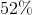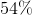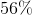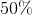Explanation:

The easiest way to count the consonants is to count the vowels and blanks first.

There are nine "A" tiles, twelve "E" tiles, nine "I" tiles, eight "O" tiles, four "U" tiles, and two "blanks". This is a total of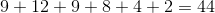tiles that are not consonants.

There are 96 tiles left after the removal of the "J", the "Q", the "X", and the "Z". Therefore, the number of consonants remaining is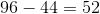, which is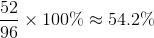This rounds to 54%.

1 3 Next →

### All SSAT Upper Level Math Resources